Last time I introduced natural transformations, and I think it's important to solve a bunch more puzzles to get a feel for what they're like. First I'll remind you of the basic definitions. I'll go through 'em quickly and informally: **Definition.** A **category** \$$\mathcal{C}\$$ consists of: 1. a collection of **objects** and 2. a set of **morphisms** \$$f : x \to y\$$ from any object \$$x\$$ to any object \$$y\$$, such that: a) each pair of morphisms \$$f : x \to y\$$ and \$$g: y \to z\$$ has a **composite** \$$g \circ f : x \to z \$$ and b) each object \$$x\$$ has a morphism \$$1_x : x \to x\$$ called its **identity**, for which i) the **associative law** holds: \$$h \circ (g \circ f) = (h \circ g) \circ f\$$, and ii) the **left and right unit laws** hold: \$$1_y \circ f = f = f \circ 1_x \$$ for any morphism \$$f: x \to y\$$. A category looks like this: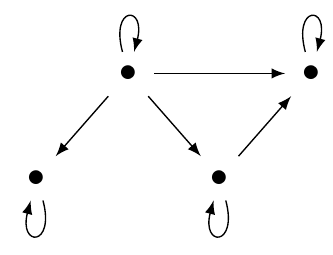**Definition.** Given categories \$$\mathcal{C}\$$ and \$$\mathcal{D}\$$, a **functor** \$$F: \mathcal{C} \to \mathcal{D} \$$ maps 1. each object \$$x\$$ of \$$\mathcal{C}\$$ to an object \$$F(x)\$$ of \$$\mathcal{D}\$$, 2. each morphism \$$f: x \to y\$$ in \$$\mathcal{C}\$$ to a morphism \$$F(f) : F(x) \to F(y) \$$ in \$$\mathcal{D}\$$ , in such a way that: a) it preserves composition: \$$F(g \circ f) = F(g) \circ F(f) \$$, and b) it preserves identities: \$$F(1_x) = 1_{F(x)}\$$. A functor looks sort of like this, leaving out some detail: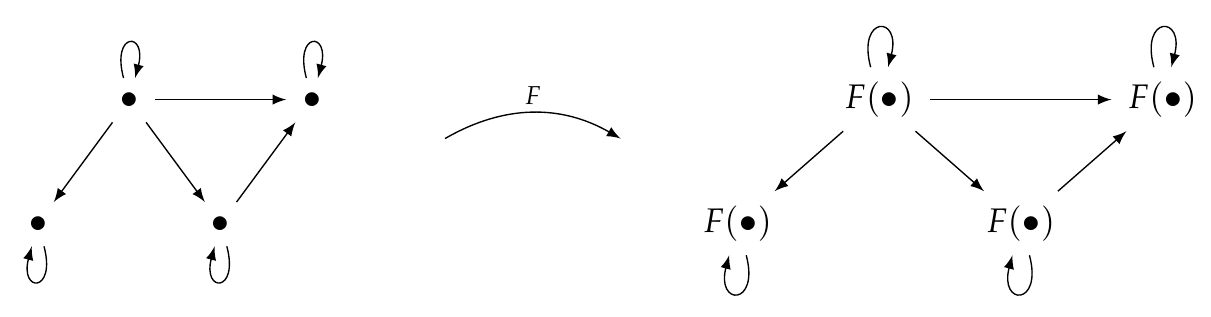**Definition.** Given categories \$$\mathcal{C},\mathcal{D}\$$ and functors \$$F, G: \mathcal{C} \to \mathcal{D}\$$, a **natural transformation** \$$\alpha : F \to G\$$ is a choice of morphism $\alpha_x : F(x) \to G(x)$ for each object \$$x \in \mathcal{C}\$$, such that for each morphism \$$f : x \to y\$$ in \$$\mathcal{C}\$$ we have $G(f) \alpha_x = \alpha_y F(f) ,$ or in other words, this **naturality square** commutes: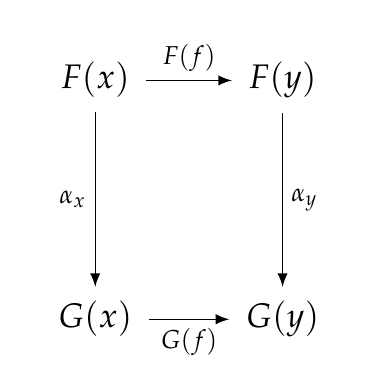A natural transformation looks sort of like this: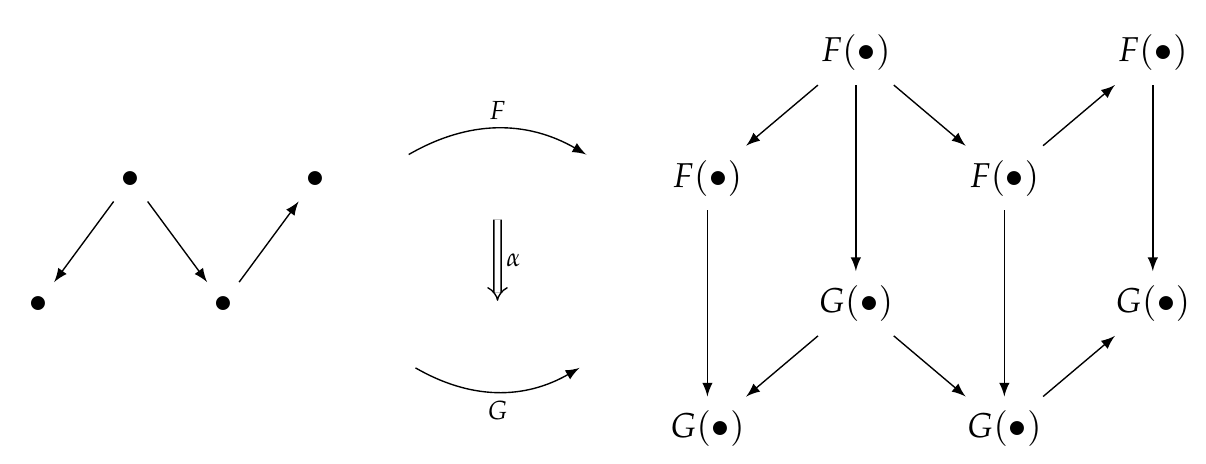You should also review the [free category on a graph](https://forum.azimuthproject.org/discussion/2204/lecture-36-categories-from-graphs/p1) if you don't remember that. Okay, now for a bunch of puzzles! If you're good at this stuff, please let beginners do the easy ones. **Puzzle 129.** Let \$$\mathbf{1}\$$ be the free category on the graph with one node and no edges: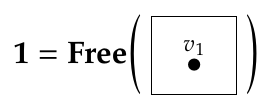Let \$$\mathbf{2}\$$ be the free category on the graph with two nodes and one edge from the first node to the second: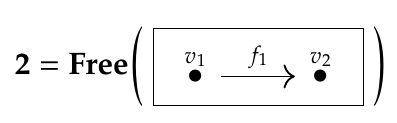How many functors are there from \$$\mathbf{1}\$$ to \$$\mathbf{2}\$$, and how many natural transformations are there between all these functors? It may help to draw a graph with functors \$$F : \mathbf{1} \to \mathbf{2} \$$ as nodes and natural transformations between these as edges. **Puzzle 130.** Let \$$\mathbf{3}\$$ be the free category on this graph: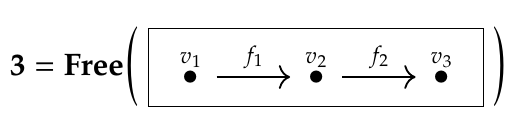How many functors are there from \$$\mathbf{1}\$$ to \$$\mathbf{3}\$$, and how many natural transformations are there between all these functors? Again, it may help to draw a graph showing all these functors and natural transformations. **Puzzle 131.** How many functors are there from \$$\mathbf{2}\$$ to \$$\mathbf{3}\$$, and how many natural transformations are there between all these functors? Again, it may help to draw a graph. **Puzzle 132.** For any category \$$\mathcal{C}\$$, what's another name for a functor \$$F: \mathbf{1} \to \mathcal{C}\$$? There's a simple answer using concepts you've already learned in this course. **Puzzle 133.** For any category \$$\mathcal{C}\$$, what's another name for a functor \$$F: \mathbf{2} \to \mathcal{C}\$$? Again, there's a simple answer using concepts you've already learned here. **Puzzle 134.** For any category \$$\mathcal{C}\$$, what's another name for a natural transformation \$$\alpha : F \Rightarrow G\$$ between functors \$$F,G: \mathbf{1} \to \mathcal{C}\$$? Yet again there's a simple answer using concepts you've learned here. **Puzzle 135.** For any category \$$\mathcal{C}\$$, what are functors \$$F : \mathcal{C} \to \mathbf{1} \$$ like? **Puzzle 136.** For any natural number \$$n\$$, we can define a category \$$\mathbf{n}\$$ generalizing the categories \$$\mathbf{1},\mathbf{2}\$$ and \$$\mathbf{3}\$$ above: it's the free category on a graph with nodes \$$v_1, \dots, v_n\$$ and edges \$$f_i : v_i \to v_{i+1}\$$ where \$$1 \le i < n\$$. How many functors are there from \$$\mathbf{m}\$$ to \$$\mathbf{n}\$$? **Puzzle 137.** How many natural transformations are there between all the functors from \$$\mathbf{m}\$$ to \$$\mathbf{n}\$$? I think Puzzle 137 is the hardest; here are two easy ones to help you recover: **Puzzle 138.** For any category, what are functors \$$F : \mathbf{0} \to \mathcal{C}\$$ like? **Puzzle 139.** For any category, what are functors \$$F : \mathcal{C} \to \mathbf{0} \$$ like? **[To read other lectures go here.](http://www.azimuthproject.org/azimuth/show/Applied+Category+Theory#Chapter_3)**